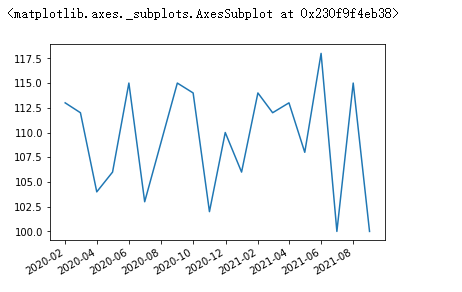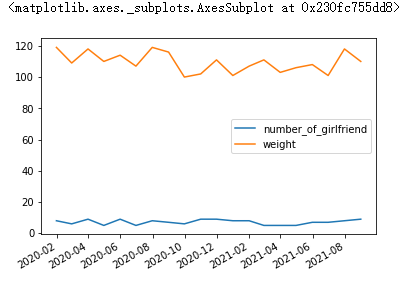# pandas中的绘图函数（线型图）

## pandas中的绘图函数

now = pd.datetime.now()
#periods 时间节点的个数
index = pd.data_range(start=now,periods=20,freq="M")
s = Series(data=np.random.randint(100,120,size=20), name="weight", index=index)
# kind='line' 设定图片 line 表示线型图
# Series 是一维数组，所以只绘制一条线
# index 作为横轴标签，values 作为数据 y轴标签的范围是values的取值范围
s.plot(kind='line')df = DataFrame(data={
"weight":np.random.randint(100,120,size=20),
'number_of_gril':np.random.randint(5,10,size=20)
},index=index)
df

# 横轴标签是index
# 纵轴标签是DataFrame所有字段的公共取值范围
# columns 是图例显示的标签，图里位置是best（整个构图中空隙最大的地方）
# DataFrame 的每一个数据成为一条线
# 如果没有设定index ，会默认填充隐示索引
df.plot(kind='line')©️2019 CSDN 皮肤主题: 护眼 设计师: 闪电赇